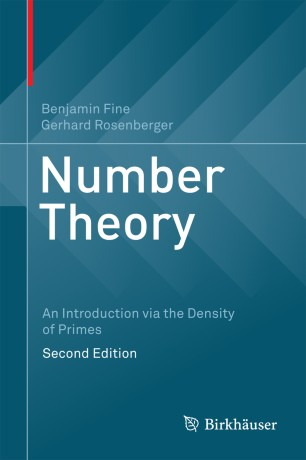# Number Theory

## An Introduction via the Density of Primes

• Benjamin Fine
• Gerhard RosenbergerTextbook

1. Front Matter
Pages i-xiii
2. Benjamin Fine, Gerhard Rosenberger
Pages 1-5
3. Benjamin Fine, Gerhard Rosenberger
Pages 7-58
4. Benjamin Fine, Gerhard Rosenberger
Pages 59-142
5. Benjamin Fine, Gerhard Rosenberger
Pages 143-218
6. Benjamin Fine, Gerhard Rosenberger
Pages 219-284
7. Benjamin Fine, Gerhard Rosenberger
Pages 285-370
8. Benjamin Fine, Gerhard Rosenberger
Pages 371-404
9. Back Matter
Pages 405-413

### Introduction

Now in its second edition, this textbook provides an introduction and overview of number theory based on the density and properties of the prime numbers. This unique approach offers both a firm background in the standard material of number theory, as well as an overview of the entire discipline. All of the essential topics are covered, such as the fundamental theorem of arithmetic, theory of congruences, quadratic reciprocity, arithmetic functions, and the distribution of primes. New in this edition are coverage of p-adic numbers, Hensel's lemma, multiple zeta-values, and elliptic curve methods in primality testing.

Key topics and features include:
• A solid introduction to analytic number theory, including full proofs of Dirichlet's Theorem and the Prime Number Theorem
• Concise treatment of algebraic number theory, including a complete presentation of primes, prime factorizations in algebraic number fields, and unique factorization of ideals
• Discussion of the AKS algorithm, which shows that primality testing is one of polynomial time, a topic not usually included in such texts
• Many interesting ancillary topics, such as primality testing and cryptography, Fermat and Mersenne numbers, and Carmichael numbers
The user-friendly style, historical context, and wide range of exercises that range from simple to quite difficult (with solutions and hints provided for select exercises) make Number Theory: An Introduction via the Density of Primes ideal for both self-study and classroom use. Intended for upper level undergraduates and beginning graduates, the only prerequisites are a basic knowledge of calculus, multivariable calculus, and some linear algebra. All necessary concepts from abstract algebra and complex analysis are introduced where needed.

### Keywords

AKS algorithm Cryptography Dirichlet's Theorem Hensel's lemma Number Theory Primality Testing Prime Number Theorem p-adic Numbers Elliptic Curve Cryptography

#### Authors and affiliations

• Benjamin Fine
• 1
• Gerhard Rosenberger
• 2
1. 1.Fairfield University Dept. MathematicsFairfieldUSA
2. 2.Universität Hamburg DortmundGermany